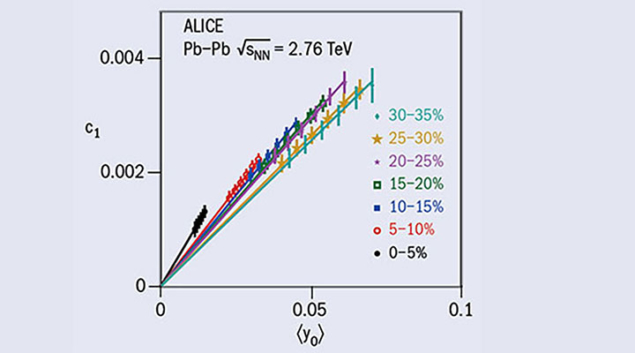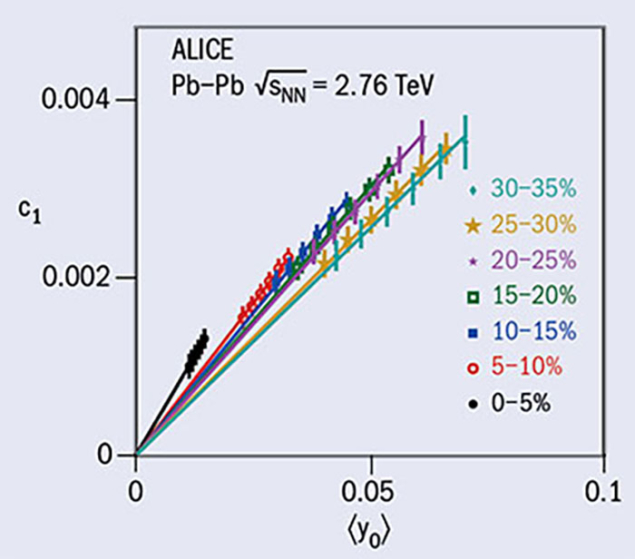# Longitudinal asymmetry tracked in heavy-ion collisions

15 January 2018Measured values of coefficient c1 as a function of estimated values of mean rapidity-shift () for different collision centralities. The lines show linear fits passing through the origin and the differences in slopes are due to changes in the width of the rapidity distributions for different centralities.

In a heavy-ion collision, a longitudinal asymmetry arises due to unequal numbers of participating nucleons from the two colliding nuclei, causing a shift in the centre-of-mass (CM) of the overlapping “participant zone” with respect to the nucleon–nucleon CM.

The asymmetry may be expressed as α = (A-B)/(A+B), where A and B are the number of nucleons participating from the two colliding nuclei. This shifts the rapidity (y0) of the participant zone with respect to the nucleon-nucleon CM rapidity, where y0 ½ ln (A/B).

First results on the asymmetry in the longitudinal direction and its effect on the pseudorapidity distributions in lead-lead collisions at a nucleon-nucleon CM energy of 2.76 TeV have been obtained with the ALICE detector, allowing investigations of the effect of variations in the initial conditions on other measurable quantities.

Since the number of participants cannot be measured directly, the asymmetry in an event was estimated by measuring the energy in the forward neutron-zero-degree-calorimeters (ZNs) in the ALICE detector. The observed distribution of asymmetry in ZNs, αzn, is used to classify events into symmetric and asymmetric by a choice of αzn. A Monte Carlo simulation using a Glauber model for the colliding nuclei is tuned to reproduce the spectrum in the ZNs and provides a relation between the measurable longitudinal asymmetry and the shift in the rapidity of the participant zone formed by the unequal number of participating nucleons.

The effect of the longitudinal asymmetry was measured on the pseudorapidity distributions of charged particles in the mid and forward regions by taking the ratio of the pseudorapidity distributions from events corresponding to different regions of asymmetry (see figure). The coefficients of a polynomial fit to the ratio characterise the effect of the asymmetry, with the coefficient of the linear term in the polynomial expansion, c1, showing a linear dependence on the mean value of y0.

This analysis confirms that the longitudinal distributions are affected by the rapidity-shift of the participant zone with respect to the nucleon-nucleon CM frame, highlighting the relevance of nucleon numbers in the production of charged particles, even at high energies. The method is potentially a new event classifier for the study of initial state fluctuations and different particle production mechanisms.